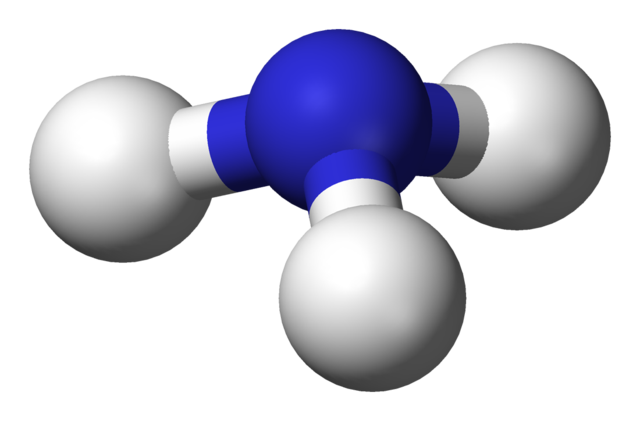Chemistry Edexcel GCSE:
Reversible Reactions

Page 1

1. When methane reacts with steam the temperature falls.

The equation for the reaction is:

CH4 + H2OCO + 3H2

What does the symbol represent, and is the reaction between methane and steam exothermic or endothermic?

 meaning of ⇌ Reaction type A equilibrium endothermic B equilibrium exothermic C Reversible reaction endothermic D Reversible reaction exothermic

Q2+3:
The following reversible reaction was investigated:

NH4Cl(s)NH3(g)  +  HCl(g)

2. What conditions are required to make this reaction go in the forward and the reverse directions?

 Forward Reverse A heat cool B heat heat C cool cool D cool heat
3. What type of reaction is the forward reaction?
• A.   neutralization
• B.   precipitation
• C.   sublimation
• D.   thermal decomposition
4. Which one of the following statements is true about reversible reactions?
• A. All reactions are reversible under lab conditions
• B. All reversible reactions are in dynamic equilibrium
• C. All reactions go to completion under lab conditions
• D. The direction of some reversible reactions can be altered by changing the reaction conditions

Q5+6:
Reversible reactions that are in dynamic equilibrium have two characteristics.

5. One of the characteristics is that the ..
• A.   forward reaction is faster than the reverse reaction
• B.   forward reaction is slower than the reverse reaction
• C.   forward and reverse reactions both stop
• D.   forward and reverse reactions occur at the same rate
6. The second characteristic is that the ..
• A.   concentrations of reactants and products are equal
• B.   concentrations of reactants and products are constant
• C.   concentration of reactants is less than concentration of products
• D.   concentration of reactants is more than concentration of products

Q7-12:
Ammonia is produced industrially in the Haber Process:

N2(g) + 3H2(g)2NH3(g)

The conditions used in the Haber Process are …7. a temperature (in degrees centigrade) of ..
8. a pressure of ..
9. a catalyst of ..
10. What is the main source of the hydrogen used in the Haber Process?
• A.   natural gas
• B.   water
• C.   air
• D.   hydrochloric acid
11. What is the main source of the nitrogen used in the Haber Process?
• A.   fertilizers
• B.   natural gas
• C.   air
• D.   coal
12. What happens to the unreacted nitrogen and hydrogen gases in the Haber Process?
• A.  they are collected and sold
• B.   they are recycled back into the reactor
• C.   they are collected and used at a later date
• D.    they are thrown away as waste products